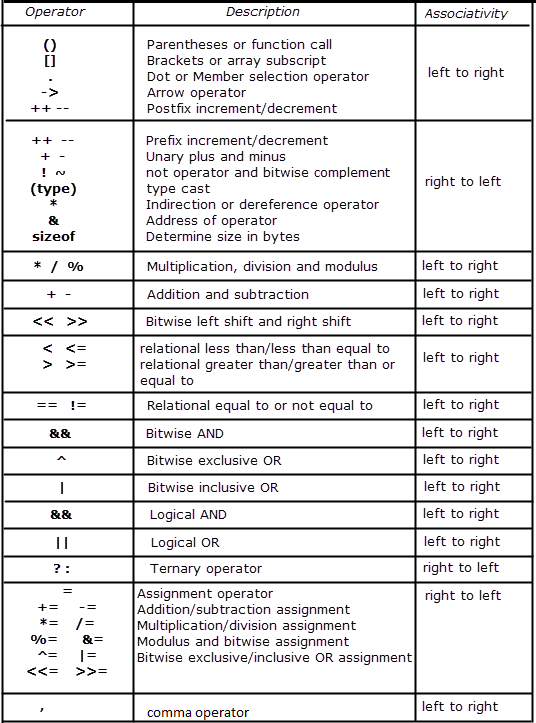# Operator Precedence And Associativity In C

If you are using more then one operator for an expression, then it is good to know the precedence and associative of the operator. The operator precedence defines the priority of the operators that means precedence indicates which operator applied first on the given expression. The higher precedence operator is evaluated before the low precedence operator.

But the problem occurs when an expression has two or more than two operators with the same precedence, so to resolve this problem a new term is introduced by the C standard, it is called associativity. The associativity defines the order in which operators of the same precedence are evaluated, that means evaluated from right to left or left to right.

#### Table of Operators with Precedence and Associativity in C

Note: In below table Precedence of operators decreases from top to bottom.Let see some examples to understand the precedence and associativity,

Example 1,

What is the output of below program?

Using the precedence table, we can calculate the output of the above described code. According the precedence table multiplication operator ( * ) has higher precedence than addition operator ( + ) and assignment operator ( = ) so multiplication will be evaluated first.

c =  a* 2 + b;
c =  5* 2 + 10;
c =  10 + 10;
c =  40;

Example 2,

What is the output of below program?

In above example, two operator ( * and / ) has the same precedence, in that situation we need to check the precedence and associativity table. According to table  *  and / has the associativity from the left to right multiplication will be evaluated first.

c = a* 2 + b / 2 ;
c = 5 * 2 + 10 / 2 ;
c = 10 + 10 / 2 ;
c = 10 + 5;
c = 15;

Note: You should always use the parentheses where more than one operator are used with expression. It prevents from the undesired behavior. See the below example,